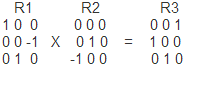# Revision history [back]

### cv::Rodrigues problem

I have two rotation matricesR1 and R2 correspond the Euler angles: (90, 0 , 0) and (0, 0, 90)

as a result, I expect to get a turn like this 90, 0 , 90

But cv::Rodrigues returns this angles : (69, 69, 69).

What I'm doing wrong

### cv::Rodrigues problem

I have two rotation matricesR1 and R2 correspond the Euler angles: (90, 0 , 0) and (0, 0, 90)

as a result, I expect to get a turn like this 90, 0 , 90

But cv::Rodrigues returns this angles : for R3: (69, 69, 69).

What I'm doing wrong